GeeksforGeeks App
Open AppBrowser
Continue

Arrays in Julia are a collection of elements just like other collections like Sets, Dictionaries, etc. Arrays are different from Sets because arrays are ordered collection of elements, and can hold duplicate values, unlike sets which require all the elements to be unique. Arrays are N-Dimensional containers divided into rows and columns. Arrays are mutable data types which mean their content can be modified, deleted, overwritten, etc.
Arrays are the heterogeneous type of containers and hence, they can hold elements of any data type. It is not mandatory to define the data type of an array before assigning the elements to the array. Julia automatically decides the data type of the array by analyzing the values assigned to it. Because of the ordered nature of an array, it makes it easier to perform operations on its values based on their index.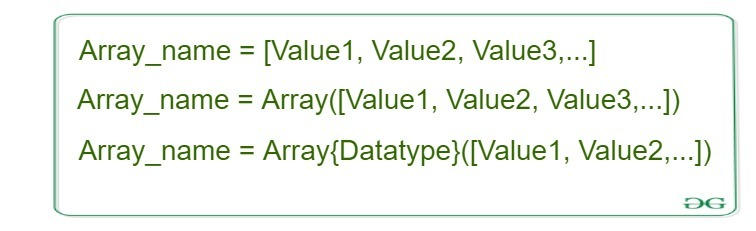### Types of Arrays

Based on their dimensions, arrays can be of three types:

• 1D Array
• 2D Array
• 3D Array

### 1D Array:

A 1D array or 1 dimensional array is a linear representation of elements. A 1D array can only have either a row or a column. It represents a type of list that can be accessed by subsequent memory locations. A 1D array in Julia is known as a Vector. Unlike other types of arrays, Vectors allow to add elements from front or back resulting in resizing the array.
Example:

```A = [1, 2, 3, 4]
4-element Array{Int64, 1}:
1
2
3
4```

### 2D Array:

A 2D array or 2 dimensional array is a representation of items in the form of rows and columns. Unlike Vectors, 2D arrays are a tabular representation of data. A 2D array in Julia is known as a Matrix. Elements in Matrix are accessed with the use of both row and column index. A 2D array can be created by removing the commas between the elements from a Vector at the time of array creation.

```A = [1 2; 3 4]

2×2 Array{Int64, 2}:
1  2
3  4```

### 3D Array:

A 3D array or 3 dimensional array is an array of arrays. A 3D array is also known as a multi-dimensional array. A 3D array is of the type NxNxN where combining arrays can be of any dimensions. A 3D array can be created with the use of cat or reshape command.
Example:

```A = cat([1 2; 3 4], [5 6; 7 8], dims=3)

2×2×2 Array{Int64, 3}:
[:, :, 1] =
1  2
3  4

[:, :, 2] =
5  6
7  8```

Here, you can give any value for ‘dims‘ and an array of that dimension will be created.

Creating an Array

An Array in Julia can be created with the use of a pre-defined keyword Array() or by simply writing array elements within square brackets([]). There are different ways of creating different types of arrays.

• A 1D array can be created by simply writing array elements within square brackets separated by commas(, ) or semicolon(;).

• A 2D array can be created by writing a set of elements without commas and then separating that set with another set of values by a semicolon.

• Similarly, a 3D array can be created by using ‘cat’ command. This command takes arrays and number of dimensions required as input and then concatenates the arrays in given dimensions.

Syntax:

```Array1 = [Value1, Value2, Value3, ...]
or
Array1 = [Value1 Value2 ; Value3 Value4]
or
Array1 = cat([Value Value; Value Value], [Value Value; Value Value], dims=3)```

## Python3

 `# Julia program to illustrate``# the use of Arrays` `# Creating a 1D array``Array1 ``=` `[``1``, ``2``, ``3``, ``4``]``println(Array1)` `# Creating a 2D array``Array2 ``=` `[``1` `2` `3``; ``4` `5` `6``]``println(Array2)` `# Creating a 3D array``# using 'cat' command``Array3 ``=` `cat([``1` `2``; ``3` `4``], [``5` `6``; ``7` `8``], [``2` `2``; ``3` `4``], dims ``=` `3``)``println(Array3)`

Output: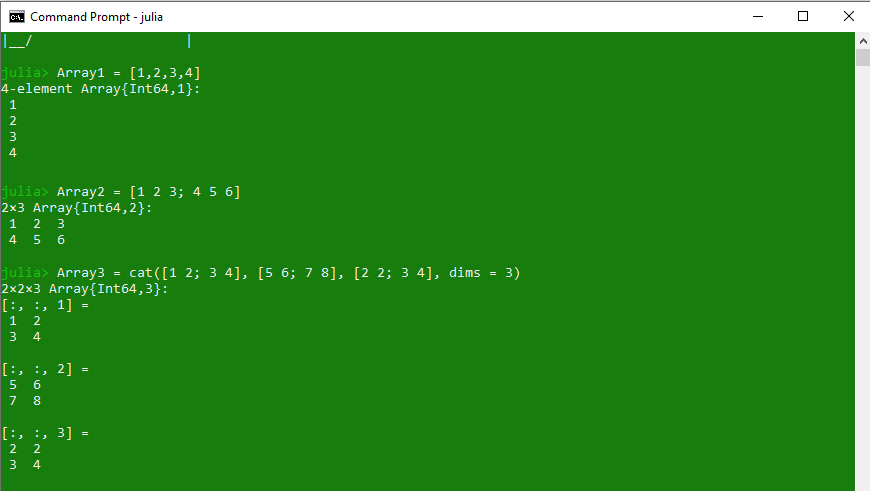Accessing Array elements

Elements of an array can be easily extracted with the use of square brackets([]). Loops can also be used to extract more than one element from an array. Also, a range of elements can also be extracted from an array by passing a range within square brackets just like Tuples.
Example: Accessing elements in a 1D array

## Python3

 `# Julia program to illustrate``# the use of Arrays` `# Creating a 1D Array``Array1 ``=` `[``1``, ``2``, ``3``, ``4``, ``"Hello"``, ``"Geeks"``]` `# Passing index value``println(Array1[``3``])` `# Accessing last value``println(Array1[end])` `# Passing a bunch of index values``println(Array1[[``3``, ``5``, ``6``]])` `# Passing a range of indices``println(Array1[``3``:end])` `# Using true-false to print values``println(Array1[[true, true, false, false, false, true]])`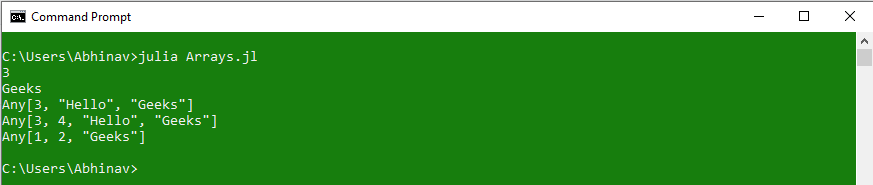Example: Accessing elements in a 2D Array

## Python3

 `# Julia program to illustrate``# the use of Arrays` `# Creating a 2D Array``Array1 ``=` `[``1` `2` `"Hello"``; ``3` `4` `"Geeks"``]` `# Passing index value``# row-column wise``println(Array1[``1``, ``3``])` `# Accessing last value``println(Array1[``2``, end])` `# Using getindex() function``println(getindex(Array1, ``2``, ``3``))` `# Using colon to indicate every row``println(Array1[:, ``3``])` `# Using colon to indicate every column``println(Array1[``1``, :])`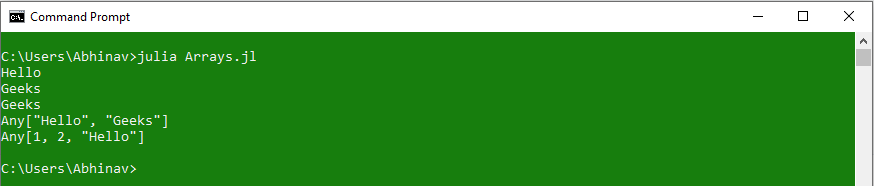Example: Accessing elements in a 3D Array

## Python3

 `# Julia program to illustrate``# the use of Arrays`` ` `# Creating a 3D array``# using 'cat' command``Array3 ``=` `cat([``1` `2``; ``3` `4``],``              ``[``"hello"` `"Geeks"``; ``"Welcome"` `"GFG"``],``              ``[``5` `6``; ``7` `8``], dims ``=` `3``)`` ` `# Accessing element using index value``println(Array3[``1``, ``2``, ``2``])`` ` `# Accessing Range of elements``println(Array3[``1``:``2``, ``1``:``2``, ``2``])`` ` `# Using colon to access every row or column``println(Array3[:, :, ``3``])`` ` `# Using getindex function``println(getindex(Array3, ``1``, ``2``, ``2``))`` ` `# Using getindex with colon operator``println(getindex(Array3, ``1``, :, ``2``))`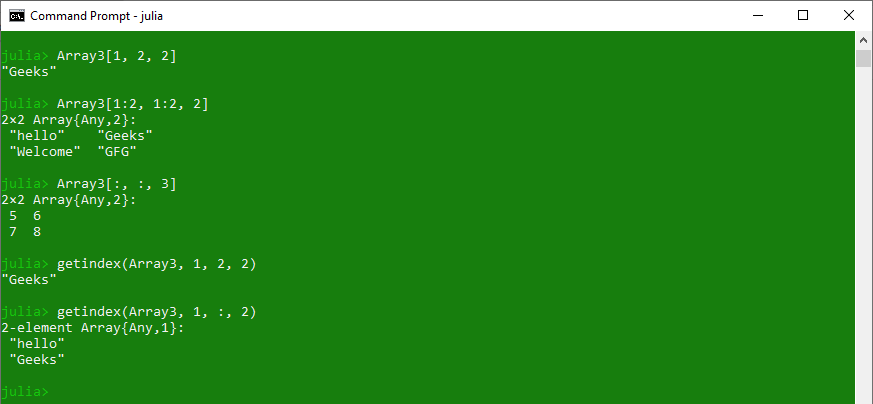Arrays are mutable type collections in Julia, hence, their values can be modified with the use of certain pre-defined keywords. Julia allows adding new elements in an array with the use of push! command. Elements in an array can also be added at a specific index by passing the range of index values in the splice! function.
Example: Adding element in 1D Array

## Python3

 `# Julia program to illustrate``# the use of Arrays` `# Creating a 1D Array``Array1 ``=` `[``1``, ``2``, ``3``, ``4``, ``"Hello"``, ``"Geeks"``]` `# Adding element at the end``push !(Array1, ``"Welcome"``)``println(Array1)` `# Adding element at the beginning``pushfirst !(Array1, ``0``)``println(Array1)` `# Adding element at specific position``splice !(Array1, ``3``:``4``, ``"GFG"``)``println(Array1)` `# Adding a range of elements``splice !(Array1, ``6``:``7``, [``1``, ``2``, ``3``])``println(Array1)`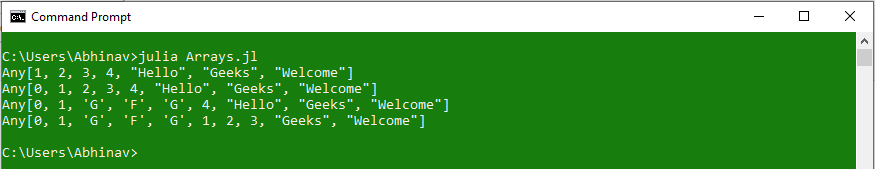Example: Adding elements in a 2D Array

## Python3

 `# Julia program to illustrate``# the use of Arrays` `# Creating a 2D Array``Array1 ``=` `[``1` `2` `"Hello"``; ``3` `4` `"Geeks"``]` `# Array of elements which are to be added``Array2 ``=` `[``5` `6` `7``; ``8` `9` `10``]` `# Appending arrays``Array3 ``=` `[Array1; Array2]``println(Array3)`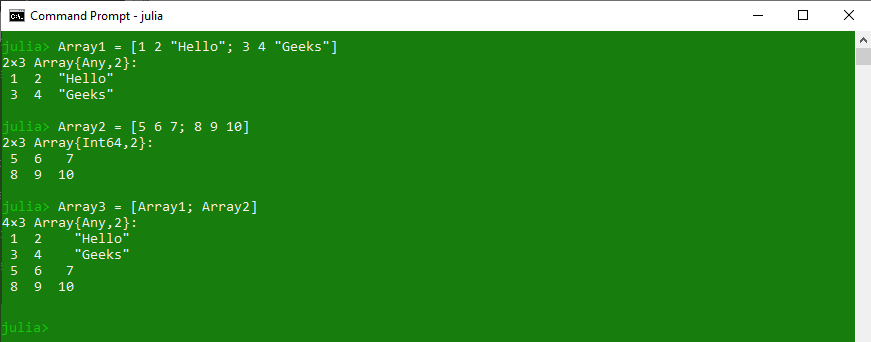Example: Adding elements in a 3D Array

## Python3

 `# Julia program to illustrate``# the use of Arrays`` ` `# Creating a 2D Array``Array1 ``=` `cat([``1` `2``; ``3` `4``],``             ``[``"hello"` `"Geeks"``; ``"Welcome"` `"GFG"``],``             ``dims ``=` `3``)`` ` `# Array of elements which is to be added``Array2 ``=` `cat([``0` `2``; ``1` `4``],``             ``[``"GFG"` `"Geeks"``; ``"abc"` `"def"` `],``             ``dims ``=` `3``)`` ` `# Appending arrays``Array3 ``=` `[Array1; Array2]``println(Array3)`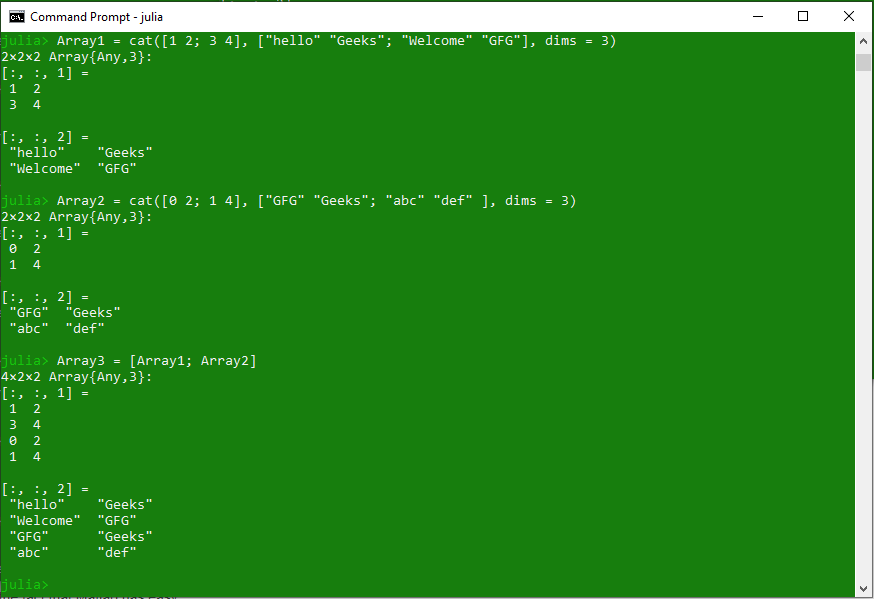Updating existing elements

Arrays in Julia are mutable and hence it allows modification of its content. Elements in arrays can be removed or updated as per requirement. To update an existing element of an array, pass the index value of that element within a square bracket.
Example:

## Python3

 `# Julia program to illustrate``# the use of Arrays`` ` `# Creating a 1D array``Array1 ``=` `[``1``, ``2``, ``3``, ``4``, ``5``, ``6``, ``"a"``, ``"b"``]``println(``"Existing 1D Array:\n"``, Array1)`` ` `# Updating value at specific index``Array1[``4``] ``=` `"Hello"``println(``"\nUpdated 1D Array:\n"``, Array1)`` ` `# Creating a 2D array``Array2 ``=` `[``1` `2` `3``; ``4` `5` `6``]``println(``"\nExisting 2D Array:\n"``, Array2)`` ` `# Updating value at specific index``Array2[``2``, ``2``] ``=` `10``println(``"\nUpdated 2D Array:\n"``, Array2)`` ` `# Creating a 3D array``Array3 ``=` `cat([``1` `2``; ``3` `4``],``             ``[``"hello"` `"Geeks"``; ``"Welcome"` `"GFG"``],``             ``dims ``=` `3``)``println(``"\nExisting 3D Array:\n"``, Array3)`` ` `# Updating value at specific index``Array3[``2``, ``2``, ``2``] ``=` `"World"``println(``"\nUpdated 3D Array:\n"``, Array3)`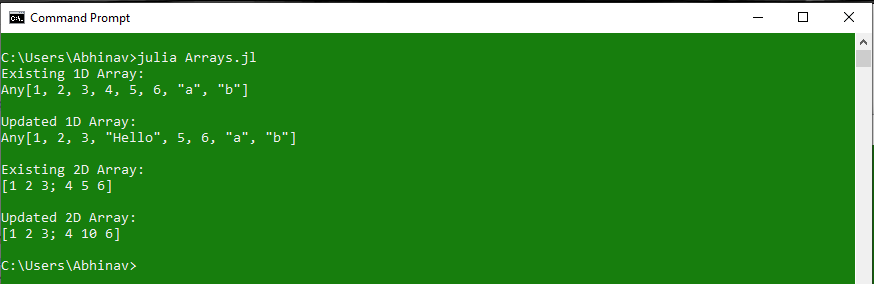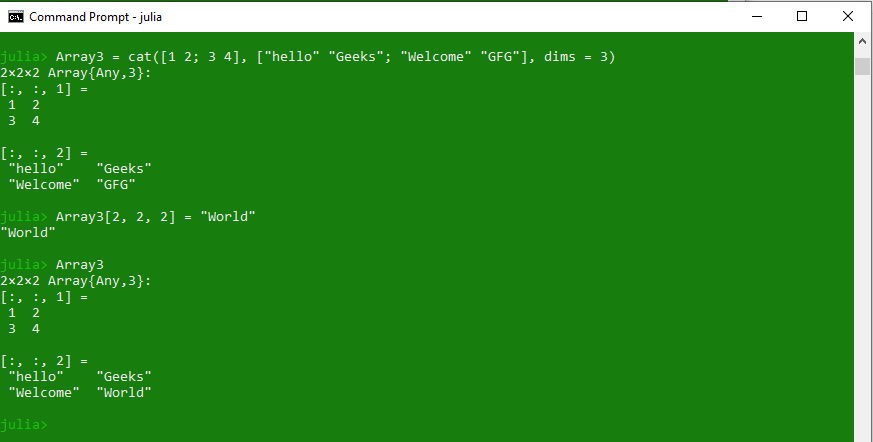Removing elements from an Array

Julia allows to delete an element from a 1D array by using predefined function pop!. This function will simply remove the last element of the array and reduce the array size by 1. To delete first element of the array, one can use popfirst! function. If there’s a need to delete an element from a specific index in the array, splice! and deleteat! functions can be used.
These methods work only on 1D array because 2D or other multidimensional arrays doesn’t allow to delete a specific element from the matrix to maintain their (row, column) order. However, one can either assign zero or undef at the place of the element to be deleted. This will not affect the order of matrix. Another way to do the same is by deleting an entire row or column.
Example: Deleting elements from 1D array

## Python3

 `# Julia program to illustrate``# the use of Arrays` `# Creating a 1D array``Array1 ``=` `[``1``, ``2``, ``3``, ``4``, ``5``, ``6``, ``"Hello"``, ``"Geeks"``]``println(``"Initial Array:\n"``, Array1)` `# Delete last element``# using pop !()``pop !(Array1)` `# Printing the array``println(``"\nArray after deleting last element:\n"``, Array1)` `# Delete first element``# using popfirst !()``popfirst !(Array1)``println(``"\nArray after deleting first element:\n"``, Array1)` `# Deleting a specific element``# using splice !()``splice !(Array1, ``4``)` `println(``"\nArray after deleting a specific(e.g. 4th) element:\n"``, Array1)` `# Deleting a range of elements``# using deleteat !()``deleteat !(Array1, ``2``:``4``)` `println(``"\nArray after deleting a range(e.g. 2:4) of elements:\n"``, Array1)` `# Deleting all the elements``# using empty !()``empty !(Array1)` `println(``"\nArray after deleting all the elements:\n"``, Array1)`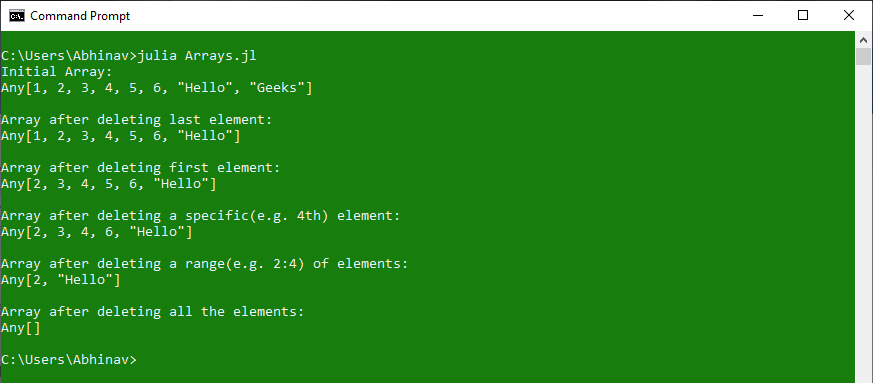Example: Deleting elements from 2D Array

## Python3

 `# Julia program to illustrate``# the use of arrays` `Array2 ``=` `[``1` `2` `3``; ``4` `5` `6``; ``"Hello"` `"Geeks"` `"World"``]``println(``"Initial Array:\n"``, Array2)` `# Assigning zero in place of``# element to be deleted(e.g. element at 1, 3)``Array2[``1``, ``3``] ``=` `0` `println(``"\nZero in place of element to be deleted:\n"``, Array2)` `# Assigning undefined value in place of``# element to be deleted(e.g. element at 2, 3)``Array2[``2``, ``3``] ``=` `undef` `println(``"\nundef in place of element to be deleted:\n"``, Array2)` `# Deleting entire row of elements(e.g. 2nd row)``Array2 ``=` `Array2[``1``:size(Array2, ``1``) .!``=` `2``, :]` `println(``"\nArray after deleting 2nd row:\n"``, Array2)` `# Deleting entire column of elements(e.g. 2nd column)``Array2 ``=` `Array2[:, ``1``:size(Array2, ``2``) .!``=` `2``]` `println(``"\nArray after deleting 2nd column:\n"``, Array2)`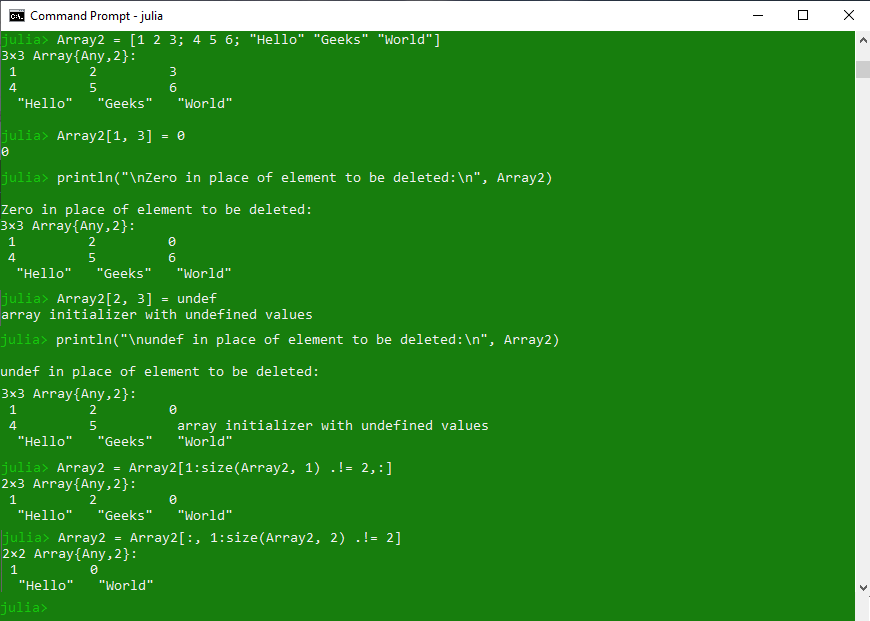In the above code, while deleting an entire row or column, we create another array of the same size and assign the elements to the new array excluding the row or column to be deleted. This is done because the deletion of a row or column from a matrix is not allowed in Julia.

Example: Deleting elements from 3D Array

## Python3

 `# Julia program to illustrate``# the use of arrays`` ` `# Creating a 3D array``Array3 ``=` `cat([``1` `2` `3``; ``3` `4` `5``; ``5` `6` `7``],``             ``[``"a"` `"b"` `"c"``; ``"c"` `"d"` `"e"``; ``"e"` `"f"` `"g"``],``             ``dims ``=` `3``)``println(``"Initial 3D Array:\n"``, Array3)`` ` `# Assigning zero in place of``# element to be deleted(e.g. element at 1, 3, 1)``Array3[``1``, ``3``, ``1``] ``=` `0`` ` `println(``"\nZero in place of element to be deleted:\n"``, Array3)`` ` `# Assigning undefined value in place of``# element to be deleted(e.g. element at 1, 3, 2)``Array3[``1``, ``3``, ``2``] ``=` `undef`` ` `println(``"\nundef in place of element to be deleted:\n"``, Array3)`` ` `# Deleting entire row of elements(e.g. 2nd row)``Array3 ``=` `Array3[``1``:size(Array3, ``2``) .!``=` `2``, :, :]`` ` `println(``"\nArray after deleting 2nd row:\n"``, Array2)`` ` `# Deleting entire column of elements(e.g. 2nd column)``Array3 ``=` `Array3[:, ``1``:size(Array3, ``2``) .!``=` `2``, :]`` ` `println(``"\nArray after deleting 2nd column:\n"``, Array2)`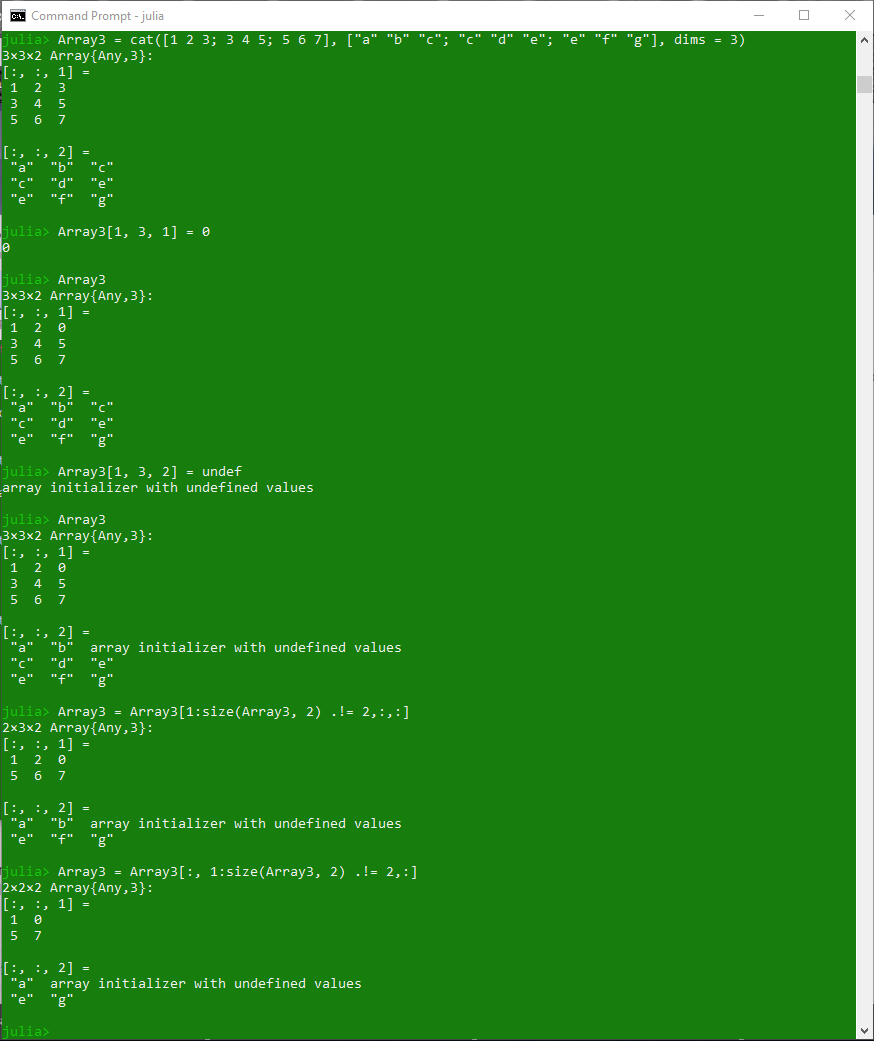#### Array Methods

.array-table {
font-family: arial, sans-serif;
border-collapse: collapse;
border: 1px solid #5fb962;
width: 100%;

}

.array-table td, th {
background-color: #c6ebd9;
border: 1px solid #5fb962;
text-align: left;

}
.array-table td:nth-child(odd) {
background-color: #c6ebd9;
}
.array-table th {
border: 1px solid #5fb962;

text-align: left;

}
.array-table td
{
border: 1px solid #5fb962;
color: black;
text-align:left !important;
}

My Personal Notes arrow_drop_up